The Australian Journal of Mathematical Analysis and Applications

 Home News Editors Volumes RGMIA Subscriptions Authors Contact

ISSN 1449-5910

Sorry death is imminent for file: ../public_html/searchroot/files/tex/v19n2/v19i2p4.tex
You searched for pare
Total of 304 results found in site

25: Paper Source PDF document

Paper's Title:

Analysis of the Flow Field in Stenosed Bifurcated Arteries Through a Mathematical Model

Author(s):

S. Chakravarty and S. Sen

Department of Mathematics, Visva-Bharati University,
Santiniketan 731235,
India
santabrata2004@yahoo.co.in

Abstract:

The present study is dealt with an appropriate mathematical model of the arotic bifurcation in the presence of constrictions using which the physiological flow field is analized. The geometry of the bifurcated arterial segment having constrictions in both the parent and its daughter arterial lumen frequently occurring in the diseased arteries causing malfunction of the cardiovascular system , is formed mathematically with the introduction of appropriate curvatures at the lateral junctions and the flow divider. The flowing blood contained in the stenosed bifurcated artery is treated to be Newtonian and the flow is considered to be two dimensional. The motion of the arterial wall and its effect on local fluid mechanics is not ruled out from the present pursuit. The flow analysis applies the time-dependent, two-dimensional incompressible nonlinear Navier-Stokes equations for Newtonian fluid. The flow field can be obtained primarily following the radial coordinate transformation and using the appropriate boundary conditions and finally adopting a suitable finite difference scheme numerically. The influences of the arterial wall distensibility and the presence of stenosis on the flow field, the flow rate and the wall shear stresses are quantified in order to indicate the susceptibility to atherosclerotic lesions and thereby to validate the applicability of the present theoretical model.

17: Paper Source PDF document

Paper's Title:

A relation between nuclear cones and full nuclear cones

Author(s):

G. Isac and A. B. Nemeth

Department of Mathematics,
P. O. Box 17000 STN Forces Kingston, Ontario,
isac-g@rmc.ca

Faculty of Mathematics and Computer Science,
Babes-Bolyai University,
3400 Cluj-Napoca,
Romania.
nemab@math.ubbcluj.ro

Abstract:

The notion of nuclear cone in locally convex spaces corresponds to the notion of well based cone in normed spaces. Using the bipolar theorem from locally convex spaces it is proved that every closed nuclear cone is a full nuclear cone. Thus every closed nuclear cone can be associated to a mapping from a family of continuous seminorms in the space to the topological dual of the space. The relation with Pareto efficiency is discussed.

11: Paper Source PDF document

Paper's Title:

Some New Generalizations of Jensen's Inequality with Related Results and Applications

Author(s):

Steven G. From

Department of Mathematics

E-mail: sfrom@unomaha.edu

Abstract:

In this paper, some new generalizations of Jensen's inequality are presented. In particular, upper and lower bounds for the Jensen gap are given and compared analytically and numerically to previously published bounds for both the discrete and continuous Jensen's inequality cases. The new bounds compare favorably to previously proposed bounds. A new method based on a series of locally linear interpolations is given and is the basis for most of the bounds given in this paper. The wide applicability of this method will be demonstrated. As by-products of this method, we shall obtain some new Hermite-Hadamard inequalities for functions which are 3-convex or 3-concave. The new method works to obtain bounds for the Jensen gap for non-convex functions as well, provided one or two derivatives of the nonlinear function are continuous. The mean residual life function of applied probability and reliability theory plays a prominent role in construction of bounds for the Jensen gap. We also present an exact integral representation for the Jensen gap in the continuous case. We briefly discuss some inequalities for other types of convexity, such as convexity in the geometric mean, and briefly discuss applications to reliability theory.

11: Paper Source PDF document

Paper's Title:

Inequalities for Discrete F-Divergence Measures: A Survey of Recent Results

Author(s):

Sever S. Dragomir1,2

1Mathematics, School of Engineering & Science
Victoria University, PO Box 14428
Melbourne City, MC 8001,
Australia
E-mail: sever.dragomir@vu.edu.au

2DST-NRF Centre of Excellence in the Mathematical and Statistical Sciences,
School of Computer Science & Applied Mathematics,
University of the Witwatersrand,
Private Bag 3, Johannesburg 2050,
South Africa
URL: http://rgmia.org/dragomir

Abstract:

In this paper we survey some recent results obtained by the author in providing various bounds for the celebrated f-divergence measure for various classes of functions f. Several techniques including inequalities of Jensen and Slater types for convex functions are employed. Bounds in terms of Kullback-Leibler Distance, Hellinger Discrimination and Varation distance are provided. Approximations of the f-divergence measure by the use of the celebrated Ostrowski and Trapezoid inequalities are obtained. More accurate approximation formulae that make use of Taylor's expansion with integral remainder are also surveyed. A comprehensive list of recent papers by several authors related this important concept in information theory is also included as an appendix to the main text.

9: Paper Source PDF document

Paper's Title:

Attempts to Define a Baum--Connes Map Via Localization of Categories for Inverse Semigroups

Author(s):

Bernhard Burgstaller

Departamento de Matematica,
CEP 88.040-900 Florianopolis-SC,
Brasil.
E-mail: bernhardburgstaller@yahoo.de
URL: http://mathematik.work/bernhardburgstaller/index.html

Abstract:

An induction functor in inverse semigroup equivariant KK-theory is considered, and together with %a restriction functors certain results similar to those known from the Mackey machinery are shown. It is also verified that for any so-called E-continuous inverse semigroup its equivariant KK-theory satisfies the universal property and is a triangulated category.

8: Paper Source PDF document

Paper's Title:

Applications of Relations and Relators in the Extensions of Stability Theorems for Homogeneous and Additive Functions

Author(s):

Árpád Száz

Institute of Mathematics, University of Debrecen,
H-4010 Debrecen, Pf. 12,
Hungary
szaz@math.klte.hu

Abstract:

By working out an appropriate technique of relations and relators and extending the ideas of the direct methods of Z. Gajda and R. Ger, we prove some generalizations of the stability theorems of D. H. Hyers, T. Aoki, Th. M. Rassias and P. Găvruţă in terms of the existence and unicity of 2-homogeneous and additive approximate selections of generalized subadditive relations of semigroups to vector relator spaces. Thus, we obtain generalizations not only of the selection theorems of Z. Gajda and R. Ger, but also those of the present author.

7: Paper Source PDF document

Paper's Title:

A New Method for Comparing Closed Intervals

Author(s):

Ibraheem Alolyan

Department of Mathematics, College of Sciences,
King Saud University, P. O. Box 2455, Riyadh 11451,
Saudi Arabia

ialolyan@ksu.edu.sa
URL:http://faculty.ksu.edu.sa/ALolyan

Abstract:

The usual ordering ``≤" on R is a total ordering, that is, for any two real numbers in R, we can determine their order without difficulty. However, for any two closed intervals in R, there is not a natural ordering among the set of all closed intervals in R. Several methods have been developed to compare two intervals. In this paper, we define the μ-ordering which is a new method for ordering closed intervals.

7: Paper Source PDF document

Paper's Title:

Euler Series Solutions for Linear Integral Equations

Author(s):

Department of Mathematics,
University of Msila 28000,
ALGERIA.
E-mail: dilmiistapha@yahoo.fr

Abstract:

In this work, we seek the approximate solution of linear integral equations by truncation Euler series approximation. After substituting the Euler expansions for the given functions of the equation and the unknown one, the equation reduces to a linear system, the solution of this latter gives the Euler coefficients and thereafter the solution of the equation. The convergence and the error analysis of this method are discussed. Finally, we compare our numerical results by others.

6: Paper Source PDF document

Paper's Title:

An Algorithm to Compute Gaussian-Type Quadrature Formulae
that Integrate Polynomials and Some Spline Functions Exactly

Author(s):

Allal Guessab

Laboratoire de Mathématiques Appliquées,
Université de Pau, 64000, Pau,
France.
allal.guessab@univ-pau.fr
URL: http://www.univ-pau.fr/~aguessab/

Abstract:

It is well-known that for sufficiently smooth integrands on an interval, numerical integration can be performed stably and efficiently via the classical (polynomial) Gauss quadrature formulae. However, for many other sets of integrands these quadrature formulae do not perform well. A very natural way of avoiding this problem is to include a wide class among arbitrary functions (not necessary polynomials) to be integrated exactly. The spline functions are natural candidates for such problems. In this paper, after studying Gaussian type quadrature formulae which are exact for spline functions and which contain boundary terms involving derivatives at both end points, we present a fast algorithm for computing their nodes and weights. It is also shown, taking advantage of the close connection with ordinary Gauss quadrature formula, that the latter are computed, via eigenvalues and eigenvectors of real symmetric tridiagonal matrices. Hence a new class of quadrature formulae can then be computed directly by standard software for ordinary Gauss quadrature formula. Comparative results with classical Gauss quadrature formulae are given to illustrate the numerical performance of the approach.

6: Paper Source PDF document

Paper's Title:

On an Autocorrection Phenomenon of the Eckhoff Interpolation

Author(s):

A. Poghosyan

Institute of Mathematics, National Academy of Sciences,
24b Marshal Baghramian ave., Yerevan 0019,
Republic of Armenia
arnak@instmath.sci.am

Abstract:

The paper considers the Krylov-Lanczos and the Eckhoff interpolations of a function with a discontinuity at a known point. These interpolations are based on certain corrections associated with jumps in the first derivatives. In the Eckhoff interpolation, approximation of the exact jumps is accomplished by the solution of a system of linear equations. We show that in the regions where the 2-periodic extension of the interpolated function is smooth, the Eckhoff interpolation converges faster compared with the Krylov-Lanczos interpolation. This accelerated convergence is known as the autocorrection phenomenon. The paper presents a theoretical explanation of this phenomenon. Numerical experiments confirm theoretical estimates.

6: Paper Source PDF document

Paper's Title:

A New Adomian Approach to Solving Integral Equations of Fredholm and Volterra Second Kind

Author(s):

Ouedraogo Seny, Nebie Abdoul Wassiha, Youssouf Pare, Blaise Some

Departement de mathematiques,
Universite Joseph Ki-Zerbo,
Burkina Faso.
E-mail: oseny@yahoo.fr, nebwass@yahoo.fr
pareyoussouf@yahoo.fr, some@univ-ouaga.bf

Abstract:

In order to simplify the resolution of Fredholm and Volterra's second type integral equations, we propose a new approach based on the Adomian Decompositional Method (ADM). We test the new approach on several examples with success.

6: Paper Source PDF document

Paper's Title:

Inequalities for Functions of Selfadjoint Operators on Hilbert Spaces:
a Survey of Recent Results

Author(s):

Sever S. Dragomir1,2

1Mathematics, College of Engineering & Science
Victoria University, PO Box 14428
Melbourne City, MC 8001,
Australia
E-mail: sever.dragomir@vu.edu.au

2DST-NRF Centre of Excellence in the Mathematical and Statistical Sciences,
School of Computer Science & Applied Mathematics,
University of the Witwatersrand,
Private Bag 3, Johannesburg 2050,
South Africa
URL: https://rgmia.org/dragomir

Abstract:

The main aim of this survey is to present recent results concerning inequalities for continuous functions of selfadjoint operators on complex Hilbert spaces. It is intended for use by both researchers in various fields of Linear Operator Theory and Mathematical Inequalities, domains which have grown exponentially in the last decade, as well as by postgraduate students and scientists applying inequalities in their specific areas.

5: Paper Source PDF document

Paper's Title:

An Easy and Efficient Way for Solving A class of Singular Two Point Boundary Value Problems

Author(s):

Muhammed I. Syam, Muhammed N. Anwar and Basem S. Attili

Mathematical Sciences Department
United Arab Emirates University, P. O. Box 17551
Al-Ain, United Arab Emirates
b.attili@uaeu.ac.ae

Abstract:

We will consider an efficient and easy way for solving a certain class of singular two point boundary value problems. We will employ the least squares method which proved to be efficient for this type of problems. Enough examples that were considered by others will be solved with comparison with the results presented there.

5: Paper Source PDF document

Paper's Title:

On Singular Numbers of Hankel Matrices of Markov Functions

Author(s):

Vasily A. Prokhorov

Department of Mathematics and Statistics,
University of South Alabama,
Mobile, Alabama 36688-0002,
USA.
E-mail: prokhoro@southalabama.edu
URL: http://www.southalabama.edu/mathstat/people/prokhorov.shtml

Abstract:

Let E ⊂ (01,1) be a compact set and let μ be a positive Borel measure with support supp μ=E. Let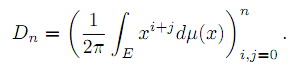In the case when E=[a,b]⊂ (-1,1) and μ satisfies the condition dμ/dx>0 a.e. on E, we investigate asymptotic behavior of singular numbers σkn,n of the Hankel matrix Dn, where kn/n→θ∈[0,1] as n→∞. Moreover, we obtain asymptotics of the Kolmogorov, Gelfand and linear k-widths, k=kn, of the unit ball An,2 of Pn∩L2(Γ) in the space L2(μ,E), where Γ={z:|z|=1} and Pn is the class of all polynomials of the degree at most n.

4: Paper Source PDF document

Paper's Title:

Reconstruction of Discontinuities of Functions Given Noisy Data

Author(s):

Eric D. Mbakop

67A Beaver Park Rd,
Framingham, MA, 01702,
U. S. A.
ericsteve86@yahoo.fr

Abstract:

Suppose one is given noisy data of a discontinuous piecewise-smooth function along with a bound on its second derivative. The locations of the points of discontinuity of f and their jump sizes are not assumed known, but are instead retrieved stably from the noisy data. The novelty of this paper is a numerical method that allows one to locate some of these points of discontinuity with an accuracy that can be made arbitrarily small.

4: Paper Source PDF document

Paper's Title:

Long Correlations Applied to the Study of Agricultural Indices in Comparison with the S&P500 index

Author(s):

M. C. Mariani, J. Libbin, M.P. Beccar Varela,
V. Kumar Mani, C. Erickson, D.J. Valles Rosales

Department of Mathematical Sciences,
Science Hall 236, New Mexico State University,
Las Cruces, NM 88003-8001,
USA.
mmariani@nmsu.edu

Abstract:

Long-time correlations in agricultural indices are studied and their behavior is compared to the well-established S&P500 index. Hurst exponent and Detrended Fluctuation Analysis (DFA) techniques are used in this analysis. We detected long-correlations in the agricultural indices and briefly discussed some features specific in comparison to the S&P500 index.

4: Paper Source PDF document

Paper's Title:

On Interpolation of L2 functions

Author(s):

Anis Rezgui

Department of Mathematics,
Faculty of Sciences,
Taibah University, Al Madina Al Munawara,
KSA.

Mathematics Department,
INSAT,
University of Carthage, Tunis,
Tunisia
E-mail: anis.rezguii@gmail.com

Abstract:

In this paper we are interested in polynomial interpolation of irregular functions namely those elements of L2(R,μ) for μ a given probability measure. This is of course doesn't make any sense unless for L2 functions that, at least, admit a continuous version. To characterize those functions we have, first, constructed, in an abstract fashion, a chain of Sobolev like subspaces of a given Hilbert space H0. Then we have proved that the chain of Sobolev like subspaces controls the existence of a continuous version for L2 functions and gives a pointwise polynomial approximation with a quite accurate error estimation.

4: Paper Source PDF document

Paper's Title:

The Dynamics of an Ebola Epidemic Model with Quarantine of Infectives

Author(s):

Eliab Horub Kweyunga

Department of Mathematics,
Kabale University,
P.O.Box 317, Kabale,
Uganda.

E-mail: hkweyunga@kab.ac.ug

Abstract:

The recurrent outbreaks of ebola in Africa present global health challenges. Ebola is a severe, very fatal disease with case fatality rates of up to 90%. In this paper, a theoretical deterministic model for ebola epidemic with quarantine of infectives is proposed and analyzed. The model exhibits two equilibria; the disease free and endemic equilibrium points. The basic reproduction number, R0, which is the main threshold, is obtained and the stability of the equilibrium points established. Using parameter values drawn from the 2014 West Africa ebola outbreak, a numerical simulation of the model is carried out. It is found that the dynamics of the model are completely determined by R0 and that a quarantine success rate of at least 70% is sufficient to contain the disease outbreak.

4: Paper Source PDF document

Paper's Title:

A Comparison Between Two Different Stochastic Epidemic Models with Respect to the Entropy

Author(s):

Department of Mathematics,
University of Sussex,
Brighton BN1 9QH,
UK.
E-mail: f.fatehi@sussex.ac.uk
URL: http://www.sussex.ac.uk/profiles/361251

Department of Pure Mathematics, Faculty of Mathematics and Computer,
Shahid Bahonar University of Kerman,
Kerman 76169-14111,
Iran.

Abstract:

In this paper at first a brief history of mathematical models is presented with the aim to clarify the reliability of stochastic models over deterministic models. Next, the necessary background about random variables and stochastic processes, especially Markov chains and the entropy are introduced. After that, entropy of SIR stochastic models is computed and it is proven that an epidemic will disappear after a long time. Entropy of a stochastic mathematical model determines the average uncertainty about the outcome of that random experiment. At the end, we introduce a chain binomial epidemic model and compute its entropy, which is then compared with the DTMC SIR epidemic model to show which one is nearer to reality.

4: Paper Source PDF document

Paper's Title:

Some Convergence Results for  Jungck-Am Iterative Process In Hyperbolic Spaces

Author(s):

Akindele Adebayo Mebawondu and Oluwatosin Temitope Mewomo

School of Mathematics, Statistics and Computer Science,
University of KwaZulu-Natal, Durban,
South Africa.
E-mail: 216028272@stu.ukzn.ac.za, mewomoo@ukzn.ac.za

Abstract:

In this paper, we introduce a new three steps iterative process called Jungck-AM iterative process and show that the proposed iterative process can be used to approximate fixed points of Jungck-contractive type mappings and Jungck-Suzuki type mappings. In addition, we establish some strong and Δ-convergence results for the approximation of fixed points of Jungck-Suzuki type mappings in the frame work of uniformly convex hyperbolic space. Furthermore, we show that the newly proposed iterative process has a better rate of convergence compare to the Jungck-Noor, Jungck-SP, Jungck-CR and some existing iterative processes in the literature. Finally, stability, data dependency results for Jungck-AM iterative process is established and we present an analytical proof and numerical examples to validate our claim.

4: Paper Source PDF document

Paper's Title:

Formulation of Approximate Mathematical Model for Incoming Water to Some Dams on Tigris and Euphrates Rivers Using Spline Function

Author(s):

Nadia M. J. Ibrahem, Heba A. Abd Al-Razak, and Muna M. Mustafa

Mathematics Department,
College of Sciences for Women,
Iraq.

Abstract:

In this paper, we formulate three mathematical models using spline functions, such as linear, quadratic and cubic functions to approximate the mathematical model for incoming water to some dams. We will implement this model on dams of both rivers; dams on the Tigris are Mosul and Amara while dams on the Euphrates are Hadetha and Al-Hindya.

4: Paper Source PDF document

Paper's Title:

Solving Two Point Boundary Value Problems by Modified Sumudu Transform Homotopy Perturbation Method

Author(s):

Asem AL Nemrat and Zarita Zainuddin

School of Mathematical Sciences,
Universiti Sains Malaysia,
11800 Penang,
Malaysia.
E-mail: alnemrata@yahoo.com
zarita@usm.my

Abstract:

This paper considers a combined form of the Sumudu transform with the modified homotopy perturbation method (MHPM) to find approximate and analytical solutions for nonlinear two point boundary value problems. This method is called the modified Sumudu transform homotopy perturbation method (MSTHPM). The suggested technique avoids the round-off errors and finds the solution without any restrictive assumptions or discretization. We will introduce an appropriate initial approximation and furthermore, the residual error will be canceled in some points of the interval (RECP). Only a first order approximation of MSTHPM will be required, as compared to STHPM, which needs more iterations for the same cases of study. After comparing figures between approximate, MSTHPM, STHPM and numerical solutions, it is found through the solutions we have obtained that they are highly accurate, indicating that the MSTHPM is very effective, simple and can be used to solve other types of nonlinear boundary value problems (BVPs).

4: Paper Source PDF document

Paper's Title:

Solving Non-Autonomous Nonlinear Systems of Ordinary Differential Equations Using Multi-Stage Differential Transform Method

Author(s):

K. A. Ahmad, Z. Zainuddin, F. A. Abdullah

School of Mathematical Sciences Universiti Sains Malaysia
11800 USM Penang
Malaysia.
zarita@usm.my
farahaini@usm.my

Abstract:

Differential equations are basic tools to describe a wide variety of phenomena in nature such as, electrostatics, physics, chemistry, economics, etc. In this paper, a technique is developed to solve nonlinear and linear systems of ordinary differential equations based on the standard Differential Transform Method (DTM) and Multi-stage Differential Transform Method (MsDTM). Comparative numerical results that we are obtained by MsDTM and Runge-Kutta method are proposed. The numerical results showed that the MsDTM gives more accurate approximation as compared to the Runge-Kutta numerical method for the solutions of nonlinear and linear systems of ordinary differential equations

4: Paper Source PDF document

Paper's Title:

Numerical Solution of Certain Types of Fredholm-Volterra Integro-Fractional Differential Equations via Bernstein Polynomials

Author(s):

Alias B. Khalaf1, Azhaar H. Sallo2 and Shazad S. Ahmed3

1Department of Mathematics, College of Science,
University of Duhok,
Kurdistan Region,
Iraq.
E-mail:  aliasbkhalaf@uod.ac

2Department of Mathematics, College of Science,
University of Duhok,
Kurdistan Region,
Iraq.
E-mail:  azhaarsallo@uod.ac

3Department of Mathematics, College of Science,
University of Sulaimani,
Kurdistan Region,
Iraq.

Abstract:

In this article we obtain a numerical solution for a certain fractional order integro-differential equations of Fredholm-Volterra type, where the fractional derivative is defined in Caputo sense. The properties of Bernstein polynomials are applied in order to convert the fractional order integro-differential equations to the solution of algebraic equations. Some numerical examples are investigated to illustrate the method. Moreover, the results obtained by this method are compared with the exact solution and with the results of some existing methods as well.

4: Paper Source PDF document

Paper's Title:

An Integration Technique for Evaluating Quadratic Harmonic Sums

Author(s):

J. M. Campbell and K.-W. Chen

Department of Mathematics and Statistics,
York University, 4700 Keele St, Toronto,
ON M3J 1P3,
E-mail: jmaxwellcampbell@gmail.com

Department of Mathematics, University of Taipei,
Taipei 10048, Taiwan.
E-mail: kwchen@uTaipei.edu.tw
URL: https://math.utaipei.edu.tw/p/412-1082-22.php

Abstract:

The modified Abel lemma on summation by parts has been applied in many ways recently to determine closed-form evaluations for infinite series involving generalized harmonic numbers with an upper parameter of two. We build upon such results using an integration technique that we apply to ``convert'' a given evaluation for such a series into an evaluation for a corresponding series involving squared harmonic numbers.

3: Paper Source PDF document

Paper's Title:

Inequalities Relating to the Gamma Function

Author(s):

Chao-Ping Chen and Feng Qi

Department of Applied Mathematics and Informatics, Research Institute of Applied Mathematics,
Henan Polytechnic University, Jiaozuo City, Henan 454000, China

chenchaoping@hpu.edu.cn

Department of Applied Mathematics and Informatics, Research Institute of Applied Mathematics,
Henan Polytechnic University, Jiaozuo City, Henan 454000, China

qifeng@hpu.edu.cn, fengqi618@member.ams.org

U
rl: http://rgmia.vu.edu.au/qi.html, http://dami.hpu.edu.cn/qifeng.html

Abstract:

For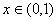, we have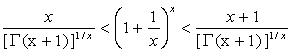.

For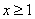,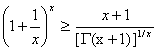,

And equality occurs for x=1.

3: Paper Source PDF document

Paper's Title:

A Study of the Effect of Density Dependence in a Matrix Population Model

Author(s):

N. Carter and M. Predescu

Department of Mathematical Sciences,
Bentley University,
Waltham, MA 02452,
U.S.A.
ncarter@bentley.edu
mpredescu@bentley.edu

Abstract:

We study the behavior of solutions of a three dimensional discrete time nonlinear matrix population model. We prove results concerning the existence of equilibrium points, boundedness, permanence of solutions, and global stability in special cases of interest. Moreover, numerical simulations are used to compare the dynamics of two main forms of the density dependence function (rational and exponential).

3: Paper Source PDF document

Paper's Title:

Optimization and Approximation for Polyhedra in Separable Hilbert Spaces

Author(s):

Paolo d'Alessandro

Department of Mathematics,
Third University of Rome,
Italy.

E-mail: pdalex45@gmail.com

Abstract:

This paper studies infinite dimensional polyhedra, covering the case in which range spaces of operators defining inequality systems are not closed. A rangespace method of linear programming is generalized to infinite dimensions and finite dimensional methods of approximation are introduced.

3: Paper Source PDF document

Paper's Title:

Strong Convergence Theorem for a Common Fixed Point of an Infinite Family of J-nonexpansive Maps with Applications

Author(s):

Charlse Ejike Chidume, Otubo Emmanuel Ezzaka and Chinedu Godwin Ezea

African University of Science and Technology,
Abuja,
Nigeria.
E-mail: cchidume@aust.edu.ng

Ebonyi State University,
Abakaliki,
Nigeria.
E-mail: mrzzaka@yahoo.com

Nnamdi Azikiwe University,
Awka,
Nigeria.
E-mail: chinedu.ezea@gmail.com

Abstract:

Let E be a uniformly convex and uniformly smooth real Banach space with dual space E*. Let {Ti}i=1 be a family of J-nonexpansive maps, where, for each i,~Ti maps E to 2E*. A new class of maps, J-nonexpansive maps from E to E*, an analogue of nonexpansive self maps of E, is introduced. Assuming that the set of common J-fixed points of {Ti}i=1 is nonempty, an iterative scheme is constructed and proved to converge strongly to a point x* in n=1FJTi. This result is then applied, in the case that E is a real Hilbert space to obtain a strong convergence theorem for approximation of a common fixed point for an infinite family of nonexpansive maps, assuming existences. The theorem obtained is compared with some important results in the literature. Finally, the technique of proof is also of independent interest.

3: Paper Source PDF document

Paper's Title:

New Implicit Kirk-Type Schemes for General Class of Quasi-Contractive Operators in Generalized Convex Metric Spaces

Author(s):

K. Rauf, O. T. Wahab and A. Ali

Department of Mathematics,
University of Ilorin, Ilorin,
Nigeria.
E-mail: krauf@unilorin.edu.ng

Department of Statistics and Mathematical Sciences,
Kwara State University, Malete,
Nigeria.

Department of Mathematics,
Mirpur University of Science and Technology, Mirpur,
Pakistan.

Abstract:

In this paper, we introduce some new implicit Kirk-type iterative schemes in generalized convex metric spaces in order to approximate fixed points for general class of quasi-contractive type operators. The strong convergence, T-stability, equivalency, data dependence and convergence rate of these results were explored. The iterative schemes are faster and better, in term of speed of convergence, than their corresponding results in the literature. These results also improve and generalize several existing iterative schemes in the literature and they provide analogues of the corresponding results of other spaces, namely: normed spaces, CAT(0) spaces and so on.

3: Paper Source PDF document

Paper's Title:

Convergence Speed of Some Random Implicit-Kirk-type Iterations for Contractive-type Random Operators

Author(s):

H. Akewe, K.S. Eke

Department of Mathematics,
Covenant University,
Canaanland, KM 10, Idiroko Road, P. M. B. 1023, Ota, Ogun State,
Nigeria.
E-mail: hudson.akewe@covenantuniversity.edu.ng, kanayo.eke@covenantuniversity.edu.ng

Abstract:

The main aim of this paper is to introduce a stochastic version of multistep type iterative scheme called a modified random implicit-Kirk multistep iterative scheme and prove strong convergence and stability results for a class of generalized contractive-type random operators. The rate of convergence of the random iterative schemes are also examined through an example. The results show that our new random implicit kirk multistep scheme perform better than other implicit iterative schemes in terms of convergence and thus have good potentials for further applications in equilibrium problems in computer science, physics and economics.

3: Paper Source PDF document

Paper's Title:

The Influence of Fluid Pressure in Macromechanical Cochlear Model

Author(s):

F. E. Aboulkhouatem1, F. Kouilily1, N. Achtaich1, N. Yousfi1 and M. El Khasmi2

1Department of Mathematics and Computer Science, Faculty of Sciences
Ben M'sik, Hassan II University, Casablanca,
Morocco.

2Department of Biology, Faculty of Sciences
Ben M'sik, Hassan II University, Casablanca,
Morocco.

E-mail: fatiaboulkhouatem@gemail.com
URL: http://www.fsb.univh2c.ma/

Abstract:

An increase of pressure in the structure of cochlea may cause a hearing loss. In this paper, we established the relationship between the fluid pressure and the amplitude of displacement of Basilar Membrane to clarify the mechanisms of hearing loss caused by increasing of this pressure. So, a mathematical cochlear model was formulated using finite difference method in order to explain and demonstrate this malfunction in passive model. Numerical simulations may be considered as helpful tools which may extend and complete the understanding of a cochlea dysfunction.

3: Paper Source PDF document

Paper's Title:

An Analytical Solution of Perturbed Fisher's Equation Using Homotopy Perturbation Method (HPM), Regular Perturbation Method (RPM) and Adomian Decomposition Method (ADM)

Author(s):

Moussa Bagayogo, Youssouf Minoungou, Youssouf Pare

Departement de Mathematique,
Universite Ouaga I Pr Joseph Ki-Zerbo,
Burkina Faso.
E-mail: moussabagayogo94@gmail.com, m.youl@yahoo.fr, pareyoussouf@yahoo.fr.

Abstract:

In this paper, Homotopy Perturbation Method (HPM), Regular Pertubation Method (RPM) and Adomian decomposition Method (ADM) are applied to Fisher equation. Then, the solution yielding the given initial conditions is gained. Finally, the solutions obtained by each method are compared

3: Paper Source PDF document

Paper's Title:

Bounds on the Jensen Gap, and Implications for Mean-Concentrated Distributions

Author(s):

Xiang Gao, Meera Sitharam, Adrian E. Roitberg

Department of Chemistry, and Department of Computer & Information Science & Engineering,
University of Florida,
Gainesville, FL 32611,
USA.
E-mail: qasdfgtyuiop@gmail.com

Abstract:

This paper gives upper and lower bounds on the gap in Jensen's inequality, i.e., the difference between the expected value of a function of a random variable and the value of the function at the expected value of the random variable. The bounds depend only on growth properties of the function and specific moments of the random variable. The bounds are particularly useful for distributions that are concentrated around the mean, a commonly occurring scenario such as the average of i.i.d. samples and in statistical mechanics.

3: Paper Source PDF document

Paper's Title:

Optimization Techniques on Affine Differential Manifolds

Author(s):

Ali S Rasheed, Faik Mayah and Ahmed A H AL-Jumaili

Ministry of Higher Education and Scientific Research,
Iraq.
E-mail: ahmedhashem@gmail.com

Department of Physics, College of Sciences,
University of Wasit,
Iraq.
E-mail: faik.mayah@gmail.com

Abstract:

In addition to solid ground of Riemannian manifolds fundamentals, this article interviews some popular optimization methods on Riemannian manifolds. Several optimization problems can be better stated on manifolds rather than Euclidean space, such as interior point methods, which in turns based on self-concordant functions (logarithmic barrier functions). Optimization schemes like the steepest descent scheme, the Newton scheme, and others can be extended to Riemannian manifolds. This paper introduces some Riemannian and non-Riemannian schemes on manifolds.

3: Paper Source PDF document

Paper's Title:

Nonlinear System of Mixed Ordered Variational Inclusions Involving XOR Operation

Author(s):

Iqbal Ahmad, Abdullah and Syed Shakaib Irfan

Department of Mechanical Engineering,
College of Engineering, Qassim University
Buraidah 51452, Al-Qassim,
Saudi Arabia.

Zakir Husain Delhi College,
University of Delhi,
JLN Marg, New Delhi- 110 002,
India.
E-mail: abdullahdu@qec.edu.sa

Department of Mathematics,
Aligarh Muslim University, Aligarh,
India.
E-mail: shakaibirfan@gmail.com

Abstract:

In this work, we introduce and solve an NSMOVI frameworks system involving XOR operation with the help of a proposed iterative algorithm in real ordered positive Hilbert spaces. We discuss the existence of a solution of a considered system of inclusions involving XOR operation by applying the resolvent operator technique with XOR operation and also study the strong convergence of the sequences generated by the considered algorithm. Further, we give a numerical example in support of our considered problem which gives the grantee that all the proposed conditions of our main result are fulfilled.

3: Paper Source PDF document

Paper's Title:

Semivectorial Bilevel Optimization on Affine-Finsler-Metric Manifolds

Author(s):

Faik Mayah1, Ali S Rasheed2 and Naseif J. Al- Jawari3

1Department of Physics,
College of Sciences,
University of Wasit,
Iraq.
E-mail: faik.mayah@gmail.com

2Ministry of Higher Education and Scientific Research,
Iraq.
E-mail: ali.math2018@yahoo.com ahmedhashem@gmail.com

3Dept. of Mathematics,
College of Science,
Iraq.
E-mail: nsaif642014@yahoo.com

Abstract:

A Finsler manifold is a differential manifold together with a Finsler metric, in this paper we construct a new class of Finsler metric affine manifolds on bilevel semivectorial with optimization problems. The first steps for this purpose involve the study of bilevel optimization on affine manifolds. The bilevel programming problem can be viewed as a static version of the noncooperative, two-person game which was introduced in the context of unbalanced economic markets. Bilevel optimization is a special kind of optimization where one problem is embedded within another.

3: Paper Source PDF document

Paper's Title:

Reverse Hölder and Minkowski type integral inequalities for n functions

Author(s):

Panagiotis T. Krasopoulos and Lazhar Bougoffa

Department of Informatics, KEAO,
Electronic National Social Security Fund,
12 Patision St., 10677, Athens,
Greece.
E-mail: pan_kras@yahoo.gr
pankras@teemail.gr

Department of Mathematics,
Faculty of Science, Imam Mohammad Ibn Saud Islamic University,
Saudi Arabia.
E-mail: lbbougoffa@imamu.edu.sa
bougoffa@hotmail.com

Abstract:

We present and prove new reverse Hölder and Minkowski type integral inequalities for n functions. We compare our results with other known results from the relative literature in order to test their performance. In this respect, our theorems can be viewed as generalizations of some already known integral inequalities.

2: Paper Source PDF document

Paper's Title:

Viability Theory And Differential Lanchester Type Models For Combat.
Differential Systems.

Author(s):

G. Isac and A. Gosselin

Department Of Mathematics, Royal Military College Of Canada,
P.O. Box 17000, S
tn Forces, Kingston, Ontario, Canada K7k 7b4

URL
:

URL
:

Abstract:

In 1914, F.W. Lanchester proposed several mathematical models based on differential equations to describe combat situations . Since then, his work has been extensively modified to represent a variety of competitions including entire wars. Differential Lanchester type models have been studied from many angles by many authors in hundreds of papers and reports. Lanchester type models are used in the planning of optimal strategies, supply and tactics. In this paper, we will show how these models can be studied from a viability theory stand point. We will introduce the notion of winning cone and show that it is a viable cone for these models. In the last part of our paper we will use the viability theory of differential equations to study Lanchester type models from the optimal theory point of view.

2: Paper Source PDF document

Paper's Title:

Reverses of the CBS Integral Inequality in Hilbert Spaces and Related Results

Author(s):

I. Brnetić, S. S. Dragomir, R. Hoxha and J. Pečarić

Department of Applied Mathematics, Faculty of Electrical Engineering and Computing,
University of Zagreb, Unska 3, 10 000 Zagreb,
Croatia
andrea@zpm.fer.hr

School of Computer Science & Mathematics, Victoria University
Po Box 14428, Melbourne Vic 8001
Australia
sever.dragomir@vu.edu.au
URL:http://rgmia.vu.edu.au/dragomir

Faculty of Applied Technical Sciences, University of Prishtina,
Mother Theresa 5, 38 000 Prishtina
Kosova
razimhoxha@yahoo.com

Faculty of Textile Technology, University of Zagreb,
Pierottijeva 6, 10000 Zagreb,
Croatia
pecaric@hazu.hr

Abstract:

There are many known reverses of the Cauchy-Bunyakovsky-Schwarz (CBS) inequality in the literature. We obtain here a general integral inequality comprising some of those results and also provide other related inequalities. The discrete case, which is of interest in its own turn, is also analysed.

2: Paper Source PDF document

Paper's Title:

On the product of M-measures in l-groups

Author(s):

A. Boccuto, B. Riěcan, and A. R. Sambucini

Dipartimento di Matematica e Informatica,
via Vanvitelli, 1 I-06123 Perugia,
Italy.
boccuto@dipmat.unipg.it
URL: http://www.dipmat.unipg.it/~boccuto

Katedra Matematiky, Fakulta Prírodných Vied,
Univerzita Mateja Bela,
Tajovského, 40, Sk-97401 Banská Bystrica,
Slovakia.
riecan@fpv.umb.sk

Dipartimento di Matematica e Informatica,
via Vanvitelli, 1 I-06123 Perugia,
Italy.
matears1@unipg.it
URL: http://www.unipg.it/~matears1

Abstract:

Some extension-type theorems and compactness properties for the
product of l-group-valued M-measures are proved.

2: Paper Source PDF document

Paper's Title:

A Coincidence Theorem for Two Kakutani Maps

Author(s):

Mircea Balaj

Department of Mathematics,
Romania.

Abstract:

In this paper we prove the following theorem: Let X be a nonempty compact convex set in a locally convex Hausdorff topological vector space, D be the set of its extremal points and F,T: XX two Kakutani maps; if for each nonempty finite subset A of D and for any x ∈ coA, F (x) coA ≠ Ř, then F and T have a coincidence point. The proof of this theorem is given first in the case when X is a simplex, then when X is a polytope and finally in the general case. Several reformulations of this result are given in the last part of the paper.

2: Paper Source PDF document

Paper's Title:

A Note On The Global Behavior Of A Nonlinear System of Difference Equations

Author(s):

Norman H. Josephy, Mihaela Predescu and Samuel W. Woolford

Department of Mathematical Sciences,
Bentley University,
Waltham, MA 02452,
U.S.A.

mpredescu@bentley.edu
njosephy@bentley.edu
swoolford@bentley.edu

Abstract:

This paper deals with the global asymptotic stability character of solutions of a discrete time deterministic model proposed by Wikan and Eide in Bulletin of Mathematical Biology, 66, 2004, 1685-1704. A stochastic extension of this model is proposed and discussed. Computer simulations suggest that the dynamics of the stochastic model includes a mixture of the dynamics observed in the deterministic model.

2: Paper Source PDF document

Paper's Title:

Traub-Potra-Type Method for Set-Valued Maps

Author(s):

Ioannis K. Argyros and Saďd Hilout

Cameron University,
Department of Mathematics Sciences,
Lawton, OK 73505,
USA

iargyros@cameron.edu

URL: http://www.cameron.edu/~ioannisa/

Poitiers University,
Laboratoire de Mathematiques et Applications,
Bd. Pierre et Marie Curie, Teleport 2, B.P. 30179,
86962 Futuroscope Chasseneuil Cedex,
France

said.hilout@math.univ-poitiers.fr

http://www-math.univ-poitiers.fr/~hilout/

Abstract:

We introduce a new iterative method for approximating a locally unique solution of variational inclusions in Banach spaces by using generalized divided differences of the first order. This method extends a method considered by Traub  (in the scalar case) and by Potra  (in the Banach spaces case) for solving nonlinear equations to variational inclusions. An existence-convergence theorem and a radius of convergence are given under some conditions on divided differences operator and Lipschitz-like continuity property of set-valued mappings. The R-order of the method is equal to the unique positive root of a certain cubic equation, which is \$1.839..., and as such it compares favorably to related methods such as the Secant method which is only of order \$1.618....

2: Paper Source PDF document

Paper's Title:

New Inequalities of Mill's Ratio and Application to The Inverse Q-function Approximation

Author(s):

Pingyi Fan

Department of Electronic Engineering,
Tsinghua University, Beijing,
China

fpy@tsinghua.edu.cn

Abstract:

In this paper, we investigate the Mill's ratio estimation problem and get two new inequalities. Compared to the well known results obtained by Gordon, they becomes tighter. Furthermore, we also discuss the inverse Q-function approximation problem and present some useful results on the inverse solution. Numerical results confirm the validness of our theoretical analysis. In addition, we also present a conjecture on the bounds of inverse solution on Q-function.

2: Paper Source PDF document

Paper's Title:

On Some Constructive Method of Rational Approximation

Author(s):

Vasiliy A. Prokhorov

Department of Mathematics and Statistics
University of South Alabama
Mobile, Alabama 36688-0002,
USA.

Abstract:

We study a constructive method of rational approximation of analytic functions based on ideas of the theory of Hankel operators. Some properties of the corresponding Hankel operator are investigated. We also consider questions related to the convergence of rational approximants. Analogues of Montessus de Ballore's and Gonchars's theorems on the convergence of rows of Padé approximants are proved.

2: Paper Source PDF document

Paper's Title:

Convergence and Stability Results for New Three Step Iteration Process in Modular Spaces

Author(s):

Naresh Kumar and Renu Chugh

Department of Mathematics,
M.D. University,
Rohtak-124001, Haryana,
India.
E-mail: nks280@gmail.com
E-mail: chugh.r1@gmail.com

Abstract:

The aim of this paper is to introduce a new iteration process (5) for ρ-contraction mappings in Modular spaces. We obtain some analytical proof for convergence and stability of our iteration process (5). We show that our iteration process (5) gives faster convergence results than the leading AK iteration process (4) for contraction mappings. Moreover, a numerical example (using the Matlab Software) is presented to compare the rate of convergence for existing iteration processes with our new iteration process (5).

2: Paper Source PDF document

Paper's Title:

Kinematic Model for Magnetic Null-points in 2 Dimensions

Author(s):

Ali Khalaf Hussain Al-Hachami

Department of Mathematics,
College of Education For Pure Sciences,
Wasit University,
Iraq.
E-mail: alhachamia@uowasit.edu.iq

Abstract:

The adjacent configurations of two-dimensional magnetic null point centers are analyzed by an immediate examination about the null. The configurations are classified as either potential or non-potential. By then the non-potential cases are subdivided into three cases depending upon whether the component of current is less than, equal to or greater than a threshold current. In addition the essential structure of reconnection in 2D is examined. It unfolds that the manner by which the magnetic flux is rebuilt. In this paper, we center on the ramifications of kinematic arrangements; that is, we fathom just Maxwell's conditions and a resistive Ohm's law.

2: Paper Source PDF document

Paper's Title:

An Efficient Modification of Differential Transform Method for Solving Integral and Integro-differential Equations

Author(s):

S. Al-Ahmad, Ibrahim Mohammed Sulaiman*, and M. Mamat

Faculty of Informatics and Computing,
Universiti Sultan Zainal Abidin,
Terengganu, Besut Campus, 22200,
Malaysia.

Abstract:

In this paper, classes of integral and integro-differential equations are solved using a modified differential transform method. This proposed technique is based on differential transform method (DTM), Laplace transform (LT) procedure and Pad\'{e} approximants (PA). The proposed method which gives a good approximation for the true solution in a large region is referred to modified differential transform method (MDTM). An algorithm was developed to illustrate the flow of the proposed method. Some numerical problems are presented to check the applicability of the proposed scheme and the obtained results from the computations are compared with other existing methods to illustrates its efficiency. Numerical results have shown that the proposed MDTM method is promising compared to other existing methods for solving integral and integro-differential equations.

2: Paper Source PDF document

Paper's Title:

Numerical Approximation by the Method of Lines with Finite-volume Approach of a Solute Transport Equation in Periodic Heterogeneous Porous Medium

Author(s):

D. J. Bambi Pemba and B. Ondami

Université Marien Ngouabi,
Factuté des Sciences et Techniques,
BP 69, Brazzaville,
Congo.
E-mail: bondami@gmail.com

Abstract:

In this paper we are interested in the numerical approximation of a two-dimensional solute transport equation in heterogeneous porous media having periodic structures. It is a class of problems which has been the subject of various works in the literature, where different methods are proposed for the determination of the so-called homogenized problem. We are interested in this paper, in the direct resolution of the problem, and we use the method of lines with a finite volume approach to discretize this equation. This discretization leads to an ordinary differential equation (ODE) that we discretize by the Euler implicit scheme. Numerical experiments comparing the obtained solution and the homogenized problem solution are presented. They show that the precision and robustness of this method depend on the ratio between, the mesh size and the parameter involved in the periodic homogenization.

2: Paper Source PDF document

Paper's Title:

Construction of a Frame Multiresolution Analysis on Locally Compact Abelian Groups

Author(s):

R. Kumar and Satyapriya

Department of Mathematics,
Kirori Mal College,
University of Delhi,
Delhi,
India.
E-mail: rajkmc@gmail.com

Department of Mathematics,
University of Delhi,
Delhi,
India.
E-mail: kmc.satyapriya@gmail.com

Abstract:

The frame multiresolution analysis (FMRA) on locally compact Abelian groups has been studied and the results concerning classical MRA have been worked upon to obtain new results. All the necessary conditions, which need to be imposed on the scaling function φ to construct a wavelet frame via FMRA, have been summed up. This process of construction of FMRA has aptly been illustrated by sufficient examples.

2: Paper Source PDF document

Paper's Title:

Some Remarks On Quasinearly Subharmonic Functions

Author(s):

Mansour Kalantar

Universite Toulouse III-Paul Sabatier,
118 Route de Narbonne, 31062 Toulouse,
France.
E-mail: mansour.kalantar@math.univ-toulouse.fr, mankalantar12@yahoo.com

Abstract:

We prove some basic properties of quasi-nearly subharmonic functions and quasi-nearly subharmonic functions in the narrow sense.

2: Paper Source PDF document

Paper's Title:

Orthogonal Collocation on Finite Elements Using Quintic Hermite Basis

Author(s):

P. Singh, N. Parumasur and C. Bansilal

University of KwaZulu-Natal,
School of Mathematics Statistics and Computer Sciences,
Private Bag X54001,
Durban, 4000,
South Africa.
E-mail: singhprook@gmail.com
parumasurn1@ukzn.ac.za
christelle18@gmail.com

Abstract:

In this paper we consider the orthogonal collocation on finite elements (OCFE) method using quintic Hermite (second degree smooth) basis functions and use it to solve partial differential equations (PDEs). The method is particularly tailored to solve third order BVPS and PDEs and to handle their special solutions such as travelling waves and solitons, which typically is the case in the KdV equation. The use of quintic polynomials and collocation using Gauss points yields a stable high order superconvergent method. OCFE using quintic Hermite basis is optimal since it is computationally more efficient than collocation methods using (first degree smooth) piecewise-polynomials and more accurate than the (third degree smooth) B-splines basis. Various computational simulations are presented to demonstrate the computational efficiency and versatility of the OCFE method.

2: Paper Source PDF document

Paper's Title:

On General Class of Nonlinear Contractive Maps and their Performance Estimates

Author(s):

Olalekan Taofeek Wahab and Salaudeen Alaro Musa

Department of Mathematics and Statistics
Kwara State University, Malete
P. M. B. 1530 Ilorin,
Nigeria.
E-mail: taofeek.wahab@kwasu.edu.ng

Abstract:

This paper considers two independent general class of nonlinear contractive maps to study the existence properties of nonlinear operators with prior degenerate. The existence properties are proved in the framework of approximate fixed points with the imposition of the general class of contractive conditions in metrical convex spaces without emphasis on completeness or compactness. For computational purposes, the performance estimates and the sensitivity dependence of these conditions are obtained for the Picard operator. Practical examples are also considered to justify the validity of the conditions. The results ensure no term is lost in the operators with prior degenerate and the conditions are strictly larger class when compare with others in the literature.

2: Paper Source PDF document

Paper's Title:

Application of Chebyshev Polynomials to Volterra-Fredholm Integral Equations

Author(s):

Department of Mathematics,
Faculty of Mathematics and
Informatics,
University of Msila,
Algeria.

E-mail: aissa.lakhal@univ-msila.dz

Abstract:

The goal of this work is to examine the numerical solution of linear Volterra-Fredholm integral equations of the second kind using the first, second, third and fourth Chebyshev polynomials. Noting that, the approximate solution is given in the form of series which converges to the exact one. Numerical examples are compared with other methods, in order to prove the applicability and the efficiency of this technical.

2: Paper Source PDF document

Paper's Title:

High Order Collocation Method for the Generalized Kuramoto-Sivashinsky Equation

Author(s):

Zanele Mkhize, Nabendra Parumasur and Pravin Singh

School of Mathematics, Statistics and Computer Sciences,
University of KwaZulu-Natal,
Private Bag X 54001,
Durban 4000.
E-mail: mkhizez2@ukzn.ac.za
parumasurn1@ukzn.ac.za
singhp@ukzn.ac.za
URL: https://www.ukzn.ac.za

Abstract:

In this paper, we derive the heptic Hermite basis functions and use them as basis functions in the orthogonal collocation on finite elements (OCFE) method. We apply the method to solve the generalized Kuramoto-Sivashinsky equation. Various numerical simulations are presented to justify the computational efficiency of the proposed method.

1: Paper Source PDF document

Paper's Title:

Long Time Behavior for a Viscoelastic Problem with a Positive Definite Kernel

Author(s):

Nasser-eddine Tatar

King Fahd University of Petroleum and Mineral, Department of Mathematical Sciences,
Dhahran, 31261 Saudi Arabia

Abstract:

We study the asymptotic behavior of solutions for an integro-differential problem which arises in the theory of viscoelasticity. It is proved that solutions go to rest in an exponential manner under new assumptions on the relaxation function in the memory term. In particular, we consider a new family of kernels which are not necessarily decreasing.

1: Paper Source PDF document

Paper's Title:

Multivalued Equilibrium Problems with Trifunction

Author(s):

Etisalat College of Engineering, P.O. Box 980, Sharjah, United Arab Emirates
noor@ece.ac.ae

Abstract:

In this paper, we use the auxiliary principle technique to suggest some new classes of iterative algorithms for solving multivalued equilibrium problems with trifunction. The convergence of the proposed methods either requires partially relaxed strongly monotonicity or pseudomonotonicity. As special cases, we obtain a number of known and new results for solving various classes of equilibrium and variational inequality problems. Since multivalued equilibrium problems with trifunction include equilibrium, variational inequality and complementarity problems as specials cases, our results continue to hold for these problems.

1: Paper Source PDF document

Paper's Title:

Asymptotic Behavior of Mixed Type Functional Equations

Author(s):

J. M. Rassias

Pedagogical Department, E.E., National and Capodistrian University of Athens, Section of Mathematics And Informatics, 4, Agamemnonos Str., Aghia Paraskevi, Athens 15342,Greece
jrassias@primedu.uoa.gr
URL: http://www.primedu.uoa.gr/~jrassias/

Abstract:

In 1983 Skof  was the first author to solve the Ulam problem for additive mappings on a restricted domain. In 1998 Jung  investigated the Hyers-Ulam stability of additive and quadratic mappings on restricted domains. In this paper we improve the bounds and thus the results obtained by Jung , in 1998 and by the author , in 2002. Besides we establish new theorems about the Ulam stability of mixed type functional equations on restricted domains. Finally, we apply our recent results to the asymptotic behavior of functional equations of different types.

1: Paper Source PDF document

Paper's Title:

Positive Solutions of Evolution Operator Equations

Author(s):

Department of Applied Mathematics,
Babes-Bolyai University,
Cluj, Romania

Abstract:

Existence and localization results are derived from Krasnoselskii’s compressionexpansion fixed point theorem in cones, for operator equations in spaces of continuous functions from a compact real interval to an abstract space. The main idea, first used in , is to handle two equivalent operator forms of the equation, one of fixed point type giving the operator to which Krasnoselskii’s theorem applies and an other one of coincidence type which is used to localize a positive solution in a shell. An application is presented for a boundary value problem associated to a fourth order partial differential equation on a rectangular domain.

1: Paper Source PDF document

Paper's Title:

Multivalued Hemiequilibrium Problems

Author(s):

Mathematics Department,
COMSATS Institute of Information Technology,
Pakistan.
noormaslam@hotmail.com

Abstract:

In this paper, we introduce and study a new class of equilibrium problems, known as multivalued hemiequilibrium problems. The auxiliary principle technique is used to suggest and analyze some new classes of iterative algorithms for solving multivalued hemiequilibrium problems. The convergence of the proposed methods either requires partially relaxed strongly monotonicity or pseudomonotonicity. As special cases, we obtain a number of known and new results for solving various classes of equilibrium and variational inequality problems. Since multivalued hemiequilibrium problems include hemiequilibrium, hemivariational inequalities, variational inequalities and complementarity problems as specials cases, our results still hold for these problems.

1: Paper Source PDF document

Paper's Title:

q-Norms are Really Norms

Author(s):

H. Belbachir, M. Mirzavaziri and M. S. Moslehian

USTHB, Faculté de Mathématiques,
B.P. 32, El Alia, 16111,
Bab Ezzouar, Alger,
Algérie.
hbelbachir@usthb.dz

Department of Mathematics,
Ferdowsi University, P. O. Box 1159,
mirzavaziri@math.um.ac.ir
URL: http://www.mirzavaziri.com

Department of Mathematics,
Ferdowsi University,
P. O. Box 1159,
moslehian@ferdowsi.um.ac.ir
URL: http://www.um.ac.ir/~moslehian/

Abstract:

Replacing the triangle inequality, in the definition of a norm, by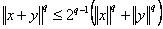, we introduce the notion of a q-norm. We establish that every q-norm is a norm in the usual sense, and that the converse is true as well.

1: Paper Source PDF document

Paper's Title:

Positive Periodic Time-Scale Solutions for Functional Dynamic Equations

Author(s):

Douglas R. Anderson and Joan Hoffacker

Department of Mathematics and Computer Science
Concordia College
andersod@cord.edu
URL:
http://www.cord.edu/faculty/andersod/

Department of Mathematical Sciences
Clemson University
Clemson, SC 29634 USA
johoff@clemson.edu
URL:
http://www.math.clemson.edu/facstaff/johoff.htm

Abstract:

Using Krasnoselskii's fixed point theorem, we establish the existence of positive periodic solutions to two pairs of related nonautonomous functional delta dynamic equations on periodic time scales, and then extend the discussion to higher-dimensional equations. Two pairs of corresponding nabla equations are also provided in an analogous manner.

1: Paper Source PDF document

Paper's Title:

Merit Functions and Error Bounds for Mixed Quasivariational Inequalities

Author(s):

Mathematics Department, COMSATS Institute of Information Technology,
noormaslam@hotmail.com

Abstract:

It is well known that the mixed quasivariational inequalities are equivalent to the fixed point problems. We use this equivalent alternative formulation to construct some merit functions for mixed quasivariational inequalities and obtain error bounds under some conditions. Since mixed quasivariational inequalities include the classical variational inequalities and the complementarity problems as special cases, our results continue to hold for these problems.

1: Paper Source PDF document

Paper's Title:

On Vector Variational Inequality Problem in Terms of Bifunctions

Author(s):

C. S. Lalitha and Monika Mehta

Department of Mathematics, Rajdhani College,
University of Delhi, Raja Garden,
Delhi 110015, India
cslalitha@rediffmail.com

Department of Mathematics, Satyawati College,
University Of Delhi, Ashok Vihar,
Phase-III, Delhi 110052, India
mridul_in@yahoo.com

Abstract:

In this paper, we consider a generalized vector variational inequality problem expressed in terms of a bifunction and establish existence theorems for this problem by using the concepts of cone convexity and cone strong quasiconvexity and employing the celebrated Fan's Lemma. We also give two types of gap functions for this problem.

1: Paper Source PDF document

Paper's Title:

Comparison Results for Solutions of Time Scale Matrix Riccati Equations and Inequalities

Author(s):

R. Hilscher

Department of Mathematical Analysis, Faculty of Science,
Masaryk University, Jan
áčkovo nám. 2a,
CZ-60200, Brno, Czech Republic.
hilscher@math.muni.cz
URL: http://www.math.muni.cz/~hilscher/

Abstract:

In this paper we derive comparison results for Hermitian solutions of time scale matrix Riccati equations and Riccati inequalities. Such solutions arise from special conjoined bases (X,U) of the corresponding time scale symplectic system via the Riccati quotient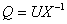. We also discuss properties of a unitary matrix solution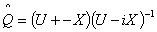of a certain associated Riccati equation.

1: Paper Source PDF document

Paper's Title:

Numerical Studies on Dynamical Systems Method for Solving Ill-posed Problems with Noise

Author(s):

N. H. Sweilam and A. M. Nagy

Mathematics Department, Faculty of Science,
Cairo University,
Giza, Egypt.
n_sweilam@yahoo.com

Mathematics Department, Faculty of Science,
Benha University,
Benha, Egypt.
abdelhameed_nagy@yahoo.com

Abstract:

In this paper, we apply the dynamical systems method proposed by A. G. RAMM, and the the variational regularization method to obtain numerical solution to some ill-posed problems with noise. The results obtained are compared to exact solutions. It is found that the dynamical systems method is preferable because it is easier to apply, highly stable, robust, and it always converges to the solution even for large size models.

1: Paper Source PDF document

Paper's Title:

Komatu Integral Transforms of Analytic Functions Subordinate to Convex Functions

Author(s):

T. N. Shanmugam and C. Ramachandran

Department of Mathematics, College of Engineering,
India
shan@annauniv.edu

Department of Mathematics, College of Engineering,
India
crjsp2004@yahoo.com

Abstract:

In this paper, we consider the class A of the functions f(z) of the form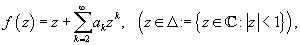which are analytic in an open disk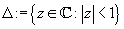and study certain subclass of the class A, for which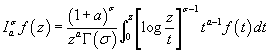has some property. Certain inclusion and the closure properties like convolution with convex univalent function etc. are studied.

1: Paper Source PDF document

Paper's Title:

On the Generalized Inverse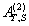over Integral Domains

Author(s):

Yaoming Yu and Guorong Wang

College of Education, Shanghai Normal University
Shanghai 200234
People's Republic of China.
yuyaoming@online.sh.cn
grwang@shnu.edu.cn

Abstract:

In this paper, we study further the generalized inverseof a matrix A over an integral domain. We give firstly some necessary and sufficient conditions for the existence of the generalized inverse, an explicit expression for the elements of the generalized inverseand an explicit expression for the generalized inverse, which reduces to the {1} inverse. Secondly, we verify that the group inverse, the Drazin inverse, the Moore-Penrose inverse and the weighted Moore-Penrose inverse are identical with the generalized inverse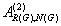for an appropriate matrix G, respectively, and then we unify the conditions for the existence and the expression for the elements of the weighted Moore-Penrose inverse, the Moore-Penrose inverse, the Drazin inverse and the group inverse over an integral domain. Thirdly, as a simple application, we give the relation between some rank equation and the existence of the generalized inverse, and a method to compute the generalized inverse. Finally, we give an example of evaluating the elements ofwithout calculating.

1: Paper Source PDF document

Paper's Title:

On the Numerical Solution for Deconvolution Problems with Noise

Author(s):

N. H. Sweilam

Cairo University, Faculty of Science, Mathematics Department,
Giza, Egypt.
n-sweilam@yahoo.com

Abstract:

In this paper three different stable methods for solving numerically deconvolution problems with noise are studied. The methods examined are the variational regularization method, the dynamical systems method, and the iterative regularized processes. Gravity surveying problem with noise is studied as a model problem. The results obtained by these methods are compared to the exact solution for the model problem. It is found that these three methods are highly stable methods and always converge to the solution even for large size models. The relative higher accuracy is obtained by using the iterative regularized processes.

1: Paper Source PDF document

Paper's Title:

On Interaction of Discontinuous Waves in a Gas with Dust Particles

Author(s):

J. Jena

Department of Mathematics, Netaji Subhas institute of technology,
Sector-3, Dwarka, New Delhi - 110 075,
India.
jjena67@rediffmail.com
jjena@nsit.ac.in

Abstract:

In this paper, the interaction of the strong shock with the weak discontinuity has been investigated for the system of partial differential equations describing one dimensional unsteady plane flow of an inviscid gas with large number of dust particles. The amplitudes of the reflected and transmitted waves after interaction of the weak discontinuity through a strong shock are evaluated by exploiting the results of general theory of wave interaction.

1: Paper Source PDF document

Paper's Title:

Construction of Lyapunov Functionals In Functional Differential Equations With Applications To Exponential Stability In Volterra Integro-differential Equations

Author(s):

Youssef N. Raffoul

Department of Mathematics, University of Dayton,
Dayton OH 45469-2316,
USA
youssef.raffoul@notes.udayton.edu

Abstract:

Non-negative definite Lyapunov functionals are employed to obtain sufficient conditions that guarantee the exponential asymptotic stability and uniform exponential asymptotic stability of the zero solution of nonlinear functional differential systems. The theory is applied to Volterra integro-differential equations in the form of proposition examples.

1: Paper Source PDF document

Paper's Title:

Normalized Truncated Levy models applied to the study of Financial Markets

Author(s):

M. C. Mariani, K. Martin, D. W. Dombrowski and D. Martinez

Department of Mathematical Sciences and Department of Finance,
New Mexico State University, P.O. Box 30001
Department 3MB Las Cruces, New Mexico 88003-8001
USA.
mmariani@nmsu.edu
kjmartin@nmsu.edu

Abstract:

This work is devoted to the study of the statistical properties of financial instruments from developed markets. We performed a new analysis of the behavior of companies corresponding to the DJIA index, and of the index itself, by using a normalized Truncated Levy walk model. We conclude that the Truncated Levy distribution describes perfectly the evolution of the companies and of the index near a crash.

1: Paper Source PDF document

Paper's Title:

On Stable Numerical Differentiation

Author(s):

N. S. Hoang and A. G. Ramm

Mathematics Department, Kansas State University
Manhattan, KS 66506-2602,
U. S. A.
nguyenhs@math.ksu.edu
ramm@math.ksu.edu
URL:http://math.ksu.edu/~ramm

Abstract:

Based on a regularized Volterra equation, two different approaches for numerical differentiation are considered. The first approach consists of solving a regularized Volterra equation while the second approach is based on solving a disretized version of the regularized Volterra equation. Numerical experiments show that these methods are efficient and compete favorably with the variational regularization method for stable calculating the derivatives of noisy functions.

1: Paper Source PDF document

Paper's Title:

The ε-Small Ball Drop Property

Author(s):

C. Donnini and A. Martellotti

Dipartimento di Statistica e Matematica per la Ricerca Economica,
Universitŕ degli Studi di Napoli "Parthenope",
Via Medina, 80133 Napoli,
Italy
chiara.donnini@uniarthenope.it

{Dipartimento di Matematica e Informatica,
Universitŕ degli Studi di Perugia,
Via Pascoli - 06123 Perugia,
Italy.
amart@dipmat.unipg.it
URL: www.dipmat.unipg.it/~amart/

Abstract:

We continue the investigation on classes of small sets in a Banach space that give alternative formulations of the Drop Property. The small sets here considered are the set having the small ball property, and we show that for sets having non-empty intrinsic core and whose affine hull contains a closed affine space of infinite dimension the Drop Property can be equivalently formulated in terms of the small ball property.

1: Paper Source PDF document

Paper's Title:

On Stan Ulam and his Mathematics

Author(s):

Krzysztof Ciesielski and Themistocles M. Rassias

Mathematics Institute, Jagiellonian University,
Ł
jasiewicza 6, 30-348 Kraków,
Poland
Department of Mathematics. National Technical University of Athens,
Zografou Campus, 15780 Athens,
Greece

Krzysztof.Ciesielski@im.uj.edu.pl
trassias@math.ntua.gr

Abstract:

In this note we give a glimpse of the curriculum vitae of Stan Ulam, his personality and some of the mathematics he was involved in.

1: Paper Source PDF document

Paper's Title:

Linearly Transformable Minimal Surfaces

Author(s):

Harold R. Parks and Walter B. Woods

Department of Mathematics, Oregon State University,
Corvallis, Oregon 97331--4605,
USA
parks@math.oregonstate.edu
URL
: http://www.math.oregonstate.edu/people/view/parks/

Abstract:

We give a complete description of a nonplanar minimal surface in R3 with the surprising property that the surface remains minimal after mapping by a linear transformation that dilates by three distinct factors in three orthogonal directions. The surface is defined in closed form using Jacobi elliptic functions.

1: Paper Source PDF document

Paper's Title:

Positive Periodic Solutions for Second-Order Differential Equations with Generalized Neutral Operator

Author(s):

Wing-Sum Cheung, Jingli Ren and Weiwei Han

Department of Mathematics,
The University of Hong Kong
Hong Kong

Department of Mathematics, Zhengzhou University
Zhengzhou 450001,
P.R. China

wscheung@hkucc.hku.hk
renjl@zzu.edu.cn

Abstract:

By some analysis of the neutral operator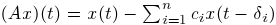and an application of the fixed-point index theorem, we obtain sufficient conditions for the existence, multiplicity and nonexistence of periodic solutions to a second-order differential equation with the prescribed neutral operator, which improve and extend some recent results of Lu-Ge, Wu-Wang, and Zhang. An example is given to illustrate our results. Moreover, the analysis of the generalized neutral operator will be helpful for other types of differential equations.

1: Paper Source PDF document

Paper's Title:

Inequalities for the Čebyšev Functional of Two Functions of Selfadjoint Operators in Hilbert Spaces

Author(s):

S. S. Dragomir

School of Engineering and Science
Victoria University, PO 14428
Melbourne City MC, Victoria 8001,
Australia

sever.dragomir@vu.edu.au
URL
: http://www.staff.vu.edu.au/RGMIA/dragomir/

Abstract:

Some recent inequalities for the Čebyšev functional of two functions of selfadjoint linear operators in Hilbert spaces, under suitable assumptions for the involved functions and operators, are surveyed.

1: Paper Source PDF document

Paper's Title:

An Improved Mesh Independence Principle for Solving Equations and their Discretizations using Newton's Method

Author(s):

Ioannis K. Argyros

Cameron university,
Department of Mathematics Sciences,
Lawton, OK 73505,
USA
iargyros@cameron.edu

Abstract:

We improve the mesh independence principle  which states that when Newton's method is applied to an equation on a Banach space as well as to their finite--dimensional discretization there is a difference of at most one between the number of steps required by the two processes to converge to within a given error tolerance. Here using a combination of Lipschitz and center Lipschitz continuity assumptions instead of just Lipschitz conditions we show that the minimum number of steps required can be at least as small as in earlier works. Some numerical examples are provided whereas our results compare favorably with earlier ones.

1: Paper Source PDF document

Paper's Title:

Topological Aspects of Scalarization in Vector Optimization Problems.

Author(s):

Peter I. Kogut, Rosanna Manzo and Igor V. Nechay

Department of Differential Equations,
Dnipropetrovsk National University, Naukova STR.,
13, 49010 Dnipropetrovsk,
Ukraine

p.kogut@i.ua

Universitŕ di Salerno,
Dipartimento di Ingegneria dell'Informazione e Matematica Applicata,
Via Ponte don Melillo, 84084 Fisciano (SA),
Italy

manzo@diima.unisa.it

Department of Technical Cybernetics,
Dnipropetrovsk Technical University,
Acad. Lazarjan STR., 2, 49010 Dnipropetrovsk,
Ukraine

i.nechay@i.ua

Abstract:

In this paper, we study vector optimization problems in partially ordered Banach spaces. We suppose that the objective mapping possesses a weakened property of lower semicontinuity and make no assumptions on the interior of the ordering cone. We derive sufficient conditions for existence of efficient solutions of the above problems and discuss the role of topological properties of the objective space. We discuss the scalarization of vector optimization problems when the objective functions are vector-valued mappings with a weakened property of lower semicontinuity. We also prove the existence of the so-called generalized efficient solutions via the scalarization process. All principal notions and assertions are illustrated by numerous examples.

1: Paper Source PDF document

Paper's Title:

Maximal Inequalities for Multidimensionally Indexed Demimartingales and the Hájek-Rényi Inequality for Associated Random Variables

Author(s):

Tasos C. Christofides and Milto Hadjikyriakou

Department of Mathematics and Statistics
University of Cyprus
P.O.Box 20537, Nicosia 1678, Cyprus

tasos@ucy.ac.cy
miltwh@gmail.com

Abstract:

Demimartingales and demisubmartingales introduced by Newman and
Wright (1982) generalize the notion of martingales and
submartingales respectively. In this paper we define
multidimensionally indexed demimartingales and demisubmartingales
and prove a maximal inequality for this general class of random
variables. As a corollary we obtain a Hájek-Rényi inequality
for multidimensionally indexed associated random variables, the bound of which, when reduced to the case of single index, is sharper than the bounds already known in the literature.

1: Paper Source PDF document

Paper's Title:

Hyperbolic Models Arising in the Theory of Longitudinal Vibration of Elastic Bars

Author(s):

1I. Fedotov, 1J. Marais, 1,2M. Shatalov and 1H.M. Tenkam

1Department of Mathematics and Statistics,
Tshwane University of Technology
Private Bag X6680, Pretoria 0001
South Africa.

fedotovi@tut.ac.za, julian.marais@gmail.com, djouosseutenkamhm@tut.ac.za.

2Manufacturing and Materials
Council of Scientific and Industrial Research (CSIR)
P.O. Box 395, Pretoria, 0001
South Africa.
mshatlov@csir.co.za

Abstract:

In this paper a unified approach to the derivation of families of one
dimensional hyperbolic differential equations and boundary conditions describing
the longitudinal vibration of elastic bars is outlined. The longitudinal and
lateral displacements are expressed in the form of a power series expansion in
the lateral coordinate. Equations of motion and boundary conditions are derived
using Hamilton's variational principle. Most of the well known models in this
field fall within the frames of the proposed theory, including the classical
model, and the more elaborated models proposed by by Rayleigh, Love, Bishop,
Mindlin, Herrmann and McNiven. The exact solution is presented for the
Mindlin-Herrmann case in terms of Green functions. Finally, deductions regarding
the accuracy of the models are made by comparison with the exact
Pochhammer-Chree solution for an isotropic cylinder.

1: Paper Source PDF document

Paper's Title:

Asymptotic Inequalities for the Maximum Modulus of the Derivative of a Polynomial

Author(s):

Clément Frappier

Département de Mathématiques et de Génie industriel École Polytechnique de Montréal,
C.P.~6079, succ. Centre-ville Montréal (Québec),

clement.frappier@polymtl.ca.

Abstract:

Let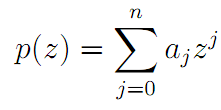be an algebraic polynomial of degree ≤n, and let ∥p∥= max {|p(z)|:|z| = 1}. We study the asymptotic behavior of the best possible constant φn,k (R), for k = 0 and k=1, in the inequality ∥p'(Rz)∥ + φn,k (R) |ak| ≤ nRn-1p∥, R → ∞.

1: Paper Source PDF document

Paper's Title:

On Generalization of Hardy-type Inequalities

Author(s):

K. Rauf, S. Ponnusamy and J. O. Omolehin

Department of Mathematics,
University of Ilorin, Ilorin,
Nigeria
krauf@unilorin.edu.ng

Department of Mathematics,
Chennai- 600 036,
India
samy@iitm.ac.in

Department of Mathematics,
University of Ilorin, Ilorin,
Nigeria
omolehin_joseph@yahoo.com

Abstract:

This paper is devoted to some new generalization of Hardy-type integral inequalities and the reversed forms. The study is to determine conditions on which the generalized inequalities hold using some known hypothesis. Improvement of some inequalities are also presented.

1: Paper Source PDF document

Paper's Title:

Szegö Limits and Haar Wavelet Basis

Author(s):

M. N. N. Namboodiri and S. Remadevi

Dept. of Mathematics, Cochin University of Science and Technology,
Cochin-21, Kerala,
India.

nambu@cusat.ac.in

Dept. of Mathematics, College of Engineering,
Cherthala, Kerala,
India.

rema@mec.ac.in

Abstract:

This paper deals with Szegö type limits for multiplication operators on L2 (R) with respect to Haar orthonormal basis. Similar studies have been carried out by Morrison for multiplication operators Tf using Walsh System and Legendre polynomials . Unlike the Walsh and Fourier basis functions, the Haar basis functions are local in nature. It is observed that Szegö type limit exist for a class of multiplication operators Tf , f∈ L (R) with respect to Haar (wavelet) system with appropriate ordering. More general classes of orderings of Haar system are identified for which the Szegö type limit exist for certain classes of multiplication operators. Some illustrative examples are also provided.

1: Paper Source PDF document

Paper's Title:

Expected Utility with Subjective Events

Author(s):

Jacob Gyntelberg and Frank Hansen

Bank for International Settlements,
Basel,
Switzerland

jacob.gyntelberg@bis.org

Tohoku University, Institute for International Education,
Sendai,
Japan

frank.hansen@m.tohoku.ac.jp

Abstract:

We provide a new theory of expected utility with subjective events modeled by a lattice of projections. This approach allows us to capture the notion of a ``small world'' as a context dependent or local state space embedded into a subjective set of events, the ``grand world''. For each situation the decision makers' subjective ``small world'' reflects the events perceived to be relevant for the act under consideration. The subjective set of events need not be representable by a classical state space. Maintaining preference axioms similar in spirit to the classical axioms, we obtain an expected utility representation which is consistent across local state spaces and separates subjective probability and utility. An added benefit is that this alternative expected utility representation allows for an intuitive distinction between risk and uncertainty.

1: Paper Source PDF document

Paper's Title:

A One-Line Derivation of the Euler and Ostrogradski Equations

Author(s):

Olivier de La Grandville

Stanford University,
Department of Management Science and Engineering,
Stanford, CA 94305,
U. S. A

Abstract:

At the very heart of major results of classical physics, the Euler and Ostrogradski equations have apparently no intuitive interpretation. In this paper we show that this is not so. Relying on Euler's initial geometric approach, we show that they can be obtained through a direct reasoning that does not imply any calculation. The intuitive approach we suggest offers two benefits: it gives immediate significance to these fundamental second-order non-linear differential equations; and second, it allows to obtain a property of the calculus of variations that does not seem to have been uncovered until now: the Euler and Ostrogradski equations can be derived not necessarily by giving a variation to the optimal function -- as is always done; one could equally well start by giving a variation to their derivative(s).

1: Paper Source PDF document

Paper's Title:

Some Functional Inequalities for the Geometric Operator Mean

Author(s):

Mustapha Raissouli

Taibah University, Faculty of Sciences, Department of Mathematics,
Al Madinah Al Munawwarah, P.O.Box 30097,
Kingdom of Saudi Arabia.

raissouli_10@hotmail.com

Abstract:

In this paper, we give some new inequalities of functional type for the power geometric operator mean involving several arguments.

1: Paper Source PDF document

Paper's Title:

Multilinear Fractional Integral Operators on Herz Spaces

Author(s):

Yasuo Komori-Furuya

School of High Technology and Human Welfare,
Tokai University, 317 Nishino Numazu Shizuoka, 410-0395
Japan

Abstract:

We prove the boundedness of the multilinear fractional integral operators of Kenig and Stein type on Herz spaces. We also show that our results are optimal.

1: Paper Source PDF document

Paper's Title:

On the Sendov Conjecture for a Root Close to the Unit Circle

Author(s):

Indraneel G. Kasmalkar

Department of Mathematics,
University of California,
Berkeley, CA 94720
United States of America

E-mail: indraneelk@berkeley.edu

Abstract:

On Sendov's conjecture, T. Chijiwa quantifies the idea stated by V. Vâjâitu and A. Zaharescu (and M. J. Miller independently), namely that if a polynomial with all roots inside the closed unit disk has a root sufficiently close to the unit circle then there is a critical point at a distance of at most one from that root. Chijiwa provides an estimate of exponential order for the required 'closeness' of the root to the unit circle so that such a critical point may exist. In this paper, we will improve this estimate to polynomial order by making major modifications and strengthening inequalities in Chijiwa's proof.

1: Paper Source PDF document

Paper's Title:

To a Banach *-algebra in a Semipartial Dynamical System

Author(s):

Bahman Tabatabaie Shourijeh and Seyed Mostafa Zebarjad

Department of Mathematics,
College of Sciences,
Shiraz University, Shiraz 71454,
Iran.

E-mail: tabataba@math.susc.ac.ir
URL: http://research.shirazu.ac.ir/faculty/More.asp?ID=207

Abstract:

By a partial dynamical system, we mean a triple containing a C*-algebra A, a discrete group G and a partial action of G on A. There are two C*--algebras associated to a given partial dynamical system. These are nothing but the certain C*-completions of a Banach *-algebra. In constructing such a Banach *-algebra, usually, a tedious limit process is used to apply. In this paper, we prove some theorems in this context without any limit process.

1: Paper Source PDF document

Paper's Title:

End-Point and Transversality Conditions in the Calculus of Variations: Derivations through Direct Reasoning

Author(s):

Olivier de La Grandville

Stanford University,
Department of Management Science and Engineering,
475 Via Ortega, Stanford, CA 94305,
U. S. A.

E-mail: ola@stanford.edu

Abstract:

We offer an intuitive explanation of the end-point and transversality conditions that complement the Euler equation in the calculus of variations. Our reasoning is based upon the fact that any variation given to an optimal function must entail a zero net gain to the functional, all consequences of implied changes in its derivative being fully taken into account.

1: Paper Source PDF document

Paper's Title:

Application of Equivalence Method to Classify Monge-Ampčre Equations of Elliptic Type

Author(s):

Moheddine Imsatfia

E-mail: imsatfia@math.jussieu.fr

Abstract:

In this paper, we apply Cartan's equivalence method to give a local classification of Monge-Ampčre equations of elliptic type. Then we find a necessary and sufficient conditions such that a Monge-Ampčre equation is either contactomorphic to the Laplace equation or to an Euler-Lagrange equation.

1: Paper Source PDF document

Paper's Title:

Weak solutions of non coercive stochastic Navier-Stokes equations in R2

Author(s):

Wilhelm Stannat and Satoshi Yokoyama

Technische Universität Berlin,
Strasse des 17. Juni 136, 10623 Berlin,
Germany.

The University of Tokyo,
Komaba, Tokyo 153-8914,
Japan.

E-mail: stannat@math.tu-berlin.de

E-mail: satoshi2@ms.u-tokyo.ac.jp

Abstract:

We prove existence of weak solutions of stochastic Navier-Stokes equations in R2 which do not satisfy the coercivity condition. The equations are formally derived from the critical point of some variational problem defined on the space of volume preserving diffeomorphisms in R2. Since the domain of our equation is unbounded, it is more difficult to get tightness of approximating sequences of solutions in comparison with the case of a bounded domain. Our approach is based on uniform a priori estimates on the enstrophy of weak solutions of the stochastic 2D-Navier-Stokes equations with periodic boundary conditions, where the periodicity is growing to infinity combined with a suitable spatial cutoff-technique.

1: Paper Source PDF document

Paper's Title:

Partial Semigroup Algebras Associated to Partial Action

Author(s):

Bahman Tabatabaie Shourijeh and Sahar Moayeri Rahni

Department of Mathematics,
College of Sciences, Shiraz University,
Shiraz, 71454,
Iran.
E-mail: tabataba@math.susc.ac.ir

Department of Mathematics,
College of Sciences, Shiraz University,
Shiraz, 71454,
Iran.
E-mail: smoayeri@shirazu.ac.ir

Abstract:

For a given inverse semigroup S, we introduce the notion of algebraic crossed product by using a given partial action of S, and we will prove that under some condition it is associative. Also we will introduce the concept of partial semigroup algebra KPar(S), and we show that the suitable quotient of KPar(S) is a kind of crossed product.

1: Paper Source PDF document

Paper's Title:

A Note on Divergent Fourier Series and λ-Permutations

Author(s):

A. Castillo, J. Chavez and H. Kim

Tufts University,
Department of Mathematics,
Medford, MA 02155,
USA
E-mail: angel.castillo@tufts.edu

Texas Tech University,
Department of Mathematics and Statistics,
Lubbock, TX 79409,
USA
E-mail: josechavez5@my.unt.edu

University of Michigan-Dearborn,
Department of Mathematics and Statistics,
Dearborn, MI 48128,
USA.
E-mail: khyejin@umich.edu

Abstract:

We present a continuous function on [-π,π] whose Fourier series diverges and it cannot be rearranged to converge by a λ-permutation.

1: Paper Source PDF document

Paper's Title:

Mapped Chebyshev Spectral Methods for Solving Second Kind Integral Equations on the Real Line

Author(s):

Ahmed Guechi and Azedine Rahmoune

Department of Mathematics, University of Bordj Bou Arréridj,
El Anasser, 34030, BBA,
Algeria.
E-mail: a.guechi2017@gmail.com
E-mail: a.rahmoune@univ-bba.dz

Abstract:

In this paper we investigate the utility of mappings to solve numerically an important class of integral equations on the real line. The main idea is to map the infinite interval to a finite one and use Chebyshev spectral-collocation method to solve the mapped integral equation in the finite interval. Numerical examples are presented to illustrate the accuracy of the method.

1: Paper Source PDF document

Paper's Title:

A Low Order Least-Squares Nonconforming Finite Element Method for Steady Magnetohydrodynamic Equations

Author(s):

Z. Yu, D. Shi and H. Zhu

College of Science,
Zhongyuan University of Technology,
Zhengzhou 450007,
China.
E-mail: 5772@zut.edu.cn

School of Mathematics and Statistics,
Zhengzhou University,
Zhengzhou 450001,
China.
E-mail: shi_dy@126.com

Mathematics Department,
University of Southern Mississippi,
Hattiesburg MS, 39406,
U.S.A
E-mail: huiqing.zhu@usm.edu

Abstract:

A low order least-squares nonconforming finite element (NFE) method is proposed for magnetohydrodynamic equations with EQ1rot element and zero-order Raviart-Thomas element. Based on the above element's typical interpolations properties, the existence and uniqueness of the approximate solutions are proved and the optimal order error estimates for the corresponding variables are derived.

1: Paper Source PDF document

Paper's Title:

An Existence of the Solution to Neutral Stochastic Functional Differential Equations Under the Holder Condition

Author(s):

Young-Ho Kim

Department of Mathematics,
Changwon National University,
Changwon, Gyeongsangnam-do 51140,
Korea.

E-mail: iyhkim@changwon.ac.kr

Abstract:

In this paper, we show the existence and uniqueness of solution of the neutral stochastic functional differential equations under weakened H\"{o}lder condition, a weakened linear growth condition, and a contractive condition. Furthermore, in order to obtain the existence of a solution to the equation we used the Picard sequence.

1: Paper Source PDF document

Paper's Title:

Global Analysis on Riemannian Manifolds

Author(s):

Louis Omenyi and Michael Uchenna

Department of Mathematics, Computer Science, Statistics and Informatics,
Alex Ekwueme Federal University, Ndufu-Alike,
Nigeria.
E-mail: omenyi.louis@funai.edu.ng, michael.uchenna@funai.edu.ng
URL: http://www.funai.edu.ng

Abstract:

In this paper, an exposition of the central concept of global analysis on a Riemannan manifold is given. We extend the theory of smooth vector fields from open subsets of Euclidean space to Riemannan manifolds. Specifically, we prove that a Riemannian manifold admits a unique solution for a system of ordinary differential equations generated by the flow of smooth tangent vectors. The idea of partial differential equations on Riemannian manifold is highlighted on the unit sphere.

1: Paper Source PDF document

Paper's Title:

Applications of Von Neumann Algebras to Rigidity Problems of (2-Step) Riemannian (Nil-)Manifolds

Author(s):

DFouman Faculty of Engineering,
College of Engineering, University of Tehran,
Iran.

Department of Mathematical Sciences,
Sharif University of Technology,
Iran
E-mail: fanai@sharif.edu

Abstract:

In this paper, basic notions of von Neumann algebra and its direct analogues in the realm of groupoids and measure spaces have been considered. By recovering the action of a locally compact Lie group from a crossed product of a von Neumann algebra, other proof of one of a geometric propositions of O'Neil and an extension of it has been proposed. Also, using the advanced exploration of nilmanifolds in measure spaces and their corresponding automorphisms (Lie algebraic derivations) a different proof of an analytic theorem of Gordon and Mao has been attained. These two propositions are of the most important ones for rigidity problems of Riemannian manifolds especially 2-step nilmanifolds.

1: Paper Source PDF document

Paper's Title:

Optimal Control Analysis of HIV/AIDS Epidemic Model with an Antiretroviral Treatment

Author(s):

U. Habibah and R. A. Sari

Mathematics Department and Reseach Group of Biomathematics,
Faculty of Mathematics and Natural Science,
Brawijaya University, Jl. Veteran Malang 65145,
Indonesia.
E-mail: ummu_habibah@ub.ac.id

Abstract:

A mathematical model of HIV/AIDS is governed by a system of ordinary differential equations in the presence of an antiretroviral treatment (ARV). The theory of optimal control is applied to an epidemic model of HIV/AIDS which an ARV is used as a control strategy in order to prevent the spread of HIV/AIDS. The optimality system is derived by applying the Pontryagin's Minimum Principle. We analyze the boundedness and positivity of solutions, and an existence of the optimal control. Numerical simulations are conducted to obtain numerical solution of the optimally system.

1: Paper Source PDF document

Paper's Title:

A New Relaxed b-metric Type and Fixed Point Results

Author(s):

P. Singh, V. Singh and Thokozani Cyprian Martin Jele

Department of Mathematics,
University of KwaZulu-Natal,
Private Bag X54001, Durban,
South Africa.
E-mail: singhp@ukzn.ac.za, singhv@ukzn.ac.za, thokozani.jele@nwu.ac.za

Abstract:

The purpose of this paper is to introduce a new relaxed α, β b-metric type by relaxing the triangle inequality. We investigate the effect that this generalization has on fixed point theorems.

1: Paper Source PDF document

Paper's Title:

Coexisting Attractors and Bubbling Route to Chaos in Modified Coupled Duffing Oscillators

Author(s):

B. Deruni1, A. S. Hacinliyan1,2, E. Kandiran3, A. C. Keles2, S. Kaouache4, M.-S. Abdelouahab4, N.-E. Hamri4

1Department of Physics,
University of Yeditepe,
Turkey.

2Department of Information Systems and Technologies,
University of Yeditepe,
Turkey

3Department of Software Development,
University of Yeditepe,
Turkey.

4Laboratory of Mathematics and their interactions,
University Center of Abdelhafid Boussouf,
Mila 43000,
Algeria.

E-mail: berc890@gmail.com
ahacinliyan@yeditepe.edu.tr
engin.kandiran@yeditepe.edu.tr
cihan.keles@yeditepe.edu.tr
s.kaouache@centr-univ-mila.dz
medsalah3@yahoo.fr
n.hamri@centre-univ-mila.dz

Abstract:

In this article dynamical behavior of coupled Duffing oscillators is analyzed under a small modification. The oscillators have cubic damping instead of linear one. Although single duffing oscillator has complex dynamics, coupled duffing systems possess a much more complex structure. The dynamical behavior of the system is investigated both numerically and analytically. Numerical results indicate that the system has double scroll attractor with suitable parameter values. On the other hand, bifurcation diagrams illustrate rich behavior of the system, and it is seen that, system enters into chaos with different routes. Beside classical bifurcations, bubbling route to chaos is observed for suitable parameter settings. On the other hand, Multistability of the system is indicated with the coexisting attractors, such that under same parameter setting the system shows different periodic and chaotic attractors. Moreover, chaotic synchronization of coupled oscillators is illustrated in final section.

1: Paper Source PDF document

Paper's Title:

Lie Group Theoretic Approach of One-Dimensional Black-Scholes Equation

Author(s):

P. L. Zondi and M. B. Matadi

Department of Mathematical sciences,
Faculty of Sciences & Agriculture, University of Zululand,
P Bag X1001, Kwa-Dlangezwa 3886,
South Africa.
zondip@unizulu.ac.za

Abstract:

This study discusses the Lie Symmetry Analysis of Black-Scholes equation via a modified local one-parameter transformations. It can be argued that the transformation of the Black-Scholes equation is firstly obtained by means of riskless rate. Thereafter, the corresponding determining equations to the reduced equation are found. Furthermore, new symmetries of the Black-Scholes equation are constructed and lead to invariant solutions.

1: Paper Source PDF document

Paper's Title:

Hankel Functional Connected to Lemniscate of Bernoulli

Author(s):

K. Ramanuja Rao, Rajnesh Lal and Kaushal Singh

Fiji National University,
Department of Mathematics & Statistics,
P.O. Box 5529, Lautoka,
Fiji.
E-mail: ramanuja.kotti@fnu.ac.fj
rajnesh.lal@fnu.ac.fj
kaushal.singh@fnu.ac.fj

Abstract:

The aim of present paper is to derive a higher bound (HB) of 3rd order Hankel determinant for a collection of holomorphic mappings connected with exactly to the right side of the lemniscate of Bernoulli, whose polar coordinates form is r2 = 2cos2(2θ). The method carried in this paper is more refined than the method adopted by the authors (see ), who worked on this problem earlier.

1: Paper Source PDF document

Paper's Title:

Trace Inequalities for Operators in Hilbert Spaces: a Survey of Recent Results

Author(s):

Sever S. Dragomir1,2

1Mathematics, School of Engineering & Science
Victoria University,
PO Box 14428 Melbourne City, MC 8001,
Australia
E-mail: sever.dragomir@vu.edu.au

2DST-NRF Centre of Excellence in the Mathematical and Statistical Sciences,
School of Computer Science & Applied Mathematics,
University of the Witwatersrand,
Private Bag 3, Johannesburg 2050,
South Africa
URL: https://rgmia.org/dragomir

Abstract:

In this paper we survey some recent trace inequalities for operators in Hilbert spaces that are connected to Schwarz's, Buzano's and Kato's inequalities and the reverses of Schwarz inequality known in the literature as Cassels' inequality and Shisha-Mond's inequality. Applications for some functionals that are naturally associated to some of these inequalities and for functions of operators defined by power series are given. Further, various trace inequalities for convex functions are presented including refinements of Jensen inequality and several reverses of Jensen's inequality. Hermite-Hadamard type inequalities and the trace version of Slater's inequality are given. Some Lipschitz type inequalities are also surveyed. Examples for fundamental functions such as the power, logarithmic, resolvent and exponential functions are provided as well.

1: Paper Source PDF document

Paper's Title:

A Caratheodory's Approximate Solutions of Stochastic Differential Equations Under the Hölder Condition

Author(s):

Bo-Kyeong Kim and Young-Ho Kim

Department of Mathematics,
Changwon National University,
Changwon, Gyeongsangnam-do 51140,
Korea.
E-mail: claire9576@naver.com
yhkim@changwon.ac.kr

Abstract:

In this paper, based on the theorem of the uniqueness of the solution of the stochastic differential equation, the convergence possibility of the Caratheodory's approximate solution was studied by approximating the unique solution. To obtain this convergence theorem, we used a Hölder condition and a weakened linear growth condition. Furthermore, The auxiliary theorems for the existence and continuity of the Caratheodory's approximate solution were investigated as a prerequisite.

1: Paper Source PDF document

Paper's Title:

Introducing the Picard-S3 Iteration for Fixed Points

Author(s):

Pravin Singh, Virath Singh and Shivani Singh

University of KwaZulu-Natal,
School of Mathematics Statistics and Computer Sciences,
Private Bag X54001,
Durban, 4000
South Africa.

Unisa,
Department of Decision Sciences,
PO Box 392,
Pretoria, 0003
South Africa.
E-mail: singhprook@gmail.com
singhv@ukzn.ac.za
shivstarsingh@gmail.com

Abstract:

In this paper we introduce a three step iteration method and show that it can be used to approximate the fixed point of a weak contraction mapping. Furthermore, we prove that this scheme is equivalent to the Mann iterative scheme. A comparison is made with other three step iterative methods by examining the speed of convergence. Results are presented in tables to support our conclusion.

1: Paper Source PDF document

Paper's Title:

D-Iterative Method for Solving a Delay Differential Equation and a Two-Point Second-Order Boundary Value Problems in Banach Spaces

Author(s):

Francis Akutsah1, Akindele Adebayo Mebawondu2, Oluwatosin Babasola3, Paranjothi Pillay4 and Ojen Kumar Narain5

1School of Mathematics,
Statistics and Computer Science,
University of KwaZulu-Natal, Durban,
South Africa.
E-mail: 216040405@stu.ukzn.ac.za, akutsah@gmail.com

2School of Mathematics,
Statistics and Computer Science,
University of KwaZulu-Natal, Durban,
South Africa.
DST-NRF Centre of Excellence in Mathematical and Statistical Sciences (CoE-MaSS),
Johannesburg,
South Africa.
Mountain Top University,
Prayer City, Ogun State,
Nigeria.
E-mail: dele@aims.ac.za

3Department of Mathematical Sciences,
University of Bath,
Claverton Down,
Bath, BA2 7AY
UK.
E-mail: ob377@bath.ac.uk

4School of Mathematics,
Statistics and Computer Science,
University of KwaZulu-Natal, Durban,
South Africa.
E-mail: pillaypi@ukzn.ac.za

5School of Mathematics,
Statistics and Computer Science,
University of KwaZulu-Natal, Durban,
South Africa.
E-mail: naraino@ukzn.ac.za

Abstract:

The purpose of this paper is to re-establish the convergence, stability and data dependence results established by  and  by removing the strong assumptions imposed on the sequences which were used to obtain their results. In addition, we introduced a modified approach using the D-iterative method to solve a two-point second-order boundary value problem, and also obtain the solution of a delay differential equations using the obtained results in this paper. The results presented in this paper do not only extend and improve the results obtained in [2, 3], it further extends and improve some existing results in the literature.

1: Paper Source PDF document

Paper's Title:

Bounds for the Extremal Eigenvalues of Positive Definite Matrices

Author(s):

Shivani Singh and Pravin Singh

Unisa, Department of Decision Sciences,
PO Box 392,
Pretoria,
0003,
South Africa.
E-mail: singhs2@unisa.ac.za

University of KwaZulu-Natal,
School of Mathematics Statistics and Computer Sciences
Private Bag X54001,
Durban,
4000,
South Africa.
E-mail: singhprook@gmail.com

Abstract:

We use a projection to achieve bounds for a vector function of the eigenvalues of a positive definite matrix. For various choices of the monotonic function we are able to obtain bounds for the extremal eigenvalues in terms of the traces of the matrix and its powers. These bounds are relatively simple to compute.

Search and serve lasted 1 second(s).